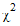# NMath User's Guide

Chapter 40. Probability Distributions (.NET, C#, CSharp, VB, Visual Basic, F#)

NMath Stats provides classes for computing the probability density function (PDF), the cumulative distribution function (CDF), the inverse cumulative distribution function, and random variable moments for a variety of probability distributions, including beta, binomial, chi-square (), exponential, F, gamma, geometric, Johnson, logistic, log-normal, negative binomial, normal (Gaussian), Poisson, Student's t, triangular, uniform, and Weibull distributions. The distribution classes share a common interface, so once you learn how to use one distribution class, it's easy to use any of the others.

This chapter describes the distribution classes and how to use them. This chapter also describes how to create correlated sets of random variables drawn from different distributions.

Top

Top